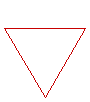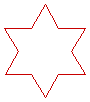Home

# Koch snowflake fractal

## What this page does

The PHP code called by the form below uses a recursive function to calculate the vertices of the Koch snowflake as a list of coordinates. For example, snowflake 1 is an equilateral triangle with three coordinate points. This list is then passed to an image-creating function in PHP which makes the PNG file you will see. The PNG opens in a separate tab or window.

 Image size (10 to 1000 pixels): Number of iterations (1 to 6):

### How does it work?

 The Koch snowflake fractal starts with an equilateral triangle.In the second stage, each side is replaced by four lines (each line one third of the original side length). Each stage after this consists of replacing every straight line with four smaller lines.This is the result of three stages.### The code

The PHP code which does this process uses recursion. This means the function at a larger scale calls itself at a smaller scale at each stage. However, once the length of each line reaches one unit, the code finally draws four lines, and the whole image appears.